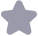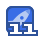### 论文研究-两种基于CVaR准则的供应链返利与惩罚契约研究.pdf收藏

1668 系统工程理论与实践 第35卷 消费者.假设市场需求D是随机的,其累积分布函数(CDF)和概率密度函数分别为F()和f(),并且F() 是非负连续的递增函数,其反函数为F-1().当产品销售期结束后.多余的产品以单位残值s进行处理不 失一般性:假设s<c<<p.为了激励零售商增加订货量,同时使整个供应链系统达到协调,制造商采用 两种不同类型的供应链返利与惩罚契约:(a)按零售商的订货量进行返利与惩罚○ orders rebate and penalty, ORP),(b)按零售商的实际销售额进行返利与惩罚( sales rebate and penalty,SRP).假设在两种契约下,制 造商设定的返利与惩罚的目标值为T,当零售商的订货量或实际销售额大于T时,制造商对超出的部分每单 位产品给予的返利为a(u>0),而当零售商的订货量或销售额小于T时,制造商对不足的部分每单位产品 给予同等的惩罚a.事件发生的顺序为 a)在销售季节到来之前,制造商向零售商提供一种返利与惩罚契约,参数为(,w,T).零售商如果接受 该契约,则进行下面的(b) (b)零售商根据自身的风险偏好程度及制造商提供的契约信息,确定产品的订货量Q,并向制造商发出 订货订单 (c)制造商按照订单安排生产计划,并在规定时间内(销售期到来之前)将产品交付给零售商 (d)销售期来临.产品实际需求D得以实现 (e)按照之前双方签订的返利与惩罚契约,制造商与零售商之间合理分配利润,转移支付完成 在分析供应链契约之前,我们先给出集中式决策下系统的总利润函数Ic(Q) IIsc(Q)=pnin(Q, D)-cQ+s(Q-D) 其中r+=max{0,x}.显然,该问题近似于经典的报童问题,当最优订货量为Qb= arg max E(Ic(Q)= F-(m=)时,系统的总利润达到最大记制造商在ORP和SRP两种契约下向零售商提供的契约参数分别 为O(,u,T)和S(.u.T).则在O(v,t,T)下,零售商与制造商的利润函数分别为 II(Q; U, u,T)=pmin(Q, D)-wQ +s(Q-D)t+u(Q-T IIm(w, u, T;Q)=(cQu Q-T 在式(2)中,零售商的利润等于销售总收益pmin(Q,D)减去采购成本Q,再加上尾货处理收益s(Q D)+与返利或惩罚收益v(Q-T).显然,当Q>T时,(Q-T)的值为正,零售商将得到返利,而当Q<T 时,u(Q-T)的值为负,零售商将受到惩罚.在式(3)中,供应商的利润等于销售利润(-c)Q减去支付给 零售商的返利总成本α(q-T)(当该值为负时,表示制造商通过惩罚零售商而得到的收益).同样地,我们可 以写出在S(v,.T)下,零售商与制造商的利润函数: II(Q; w, u, T)=pmin(Q, D)-wQ+s(Q-D)++ulmin(Q, D)-TI Im(ou, l, T: Q)=(w-c)Q-1min(Q, D)-TI 与ORP契约不同的是,SRP契约是制造商根据产品实际的销售额进行返利或惩罚,具体体现在表达式 (Q-T)与u(min(,D)-T)的区别上特别地,当Q<D时,产品实际的销售额为min(,D)=(,此时 ORP与SRP的表达式是一样的下面引入风险度量准则—条件风险价值(CVaR)的概念 12风险度量准则:CVaR 在本小节中,我们着重介绍风险度量准则CVaR的定义.假设x(Q,D)是关于库存水平和随机性需 求D的利润函数,则下式中的q(x(u,D)表示丌(μD)的m分位数 1n(丌(/,D)=nf{2|Pr(丌(4,D)≤2)≥T} CVaR风险度量准则忽略了利润超出η分位数的平均利润,主要考虑和度量了低于n分位数的平均利润, 这正是决策者关心的部分.此外,良好的计算特性已经使CVaR在金融和保险领域得到了广泛的应用.关于 n-CVar一个更一般化的定义为: CVaRn(T(u, D))=maxv+-Enin(r(u, D)-1, 0) ∈R 其中E表示期望算子,η∈(0,为风险厌恶因子,反映了决策者的风险厌恶程度,其值越小,表示决策者越 厌恶风险特别地,”=1表示决策者是风险中立的 根据 Agrawal等7,Gan等16-17对考虑风险厌恶背景下供应链协调的定义,一种契约能够协调供应 链必须满足以下三个条件:(i)制造商和零售商双方在该契约下的期望利润不能低于各自的保留利润:(i)风 第7期 李建斌,等:两种基于CVaR准则的供应链返利与惩罚契约研究 1669 险厌恶的零售商在度量准则下(如CⅤaR等)的期望利润达到最大(ⅲi)供应链的总利润实现最大化.其中, 条件()是供应链成员接受契约的前提条件,只有当双方都“有利可图”时,即在供应链契约下获得的利润都 不小于保留利润时,制造商和零售商才有“改变现状”而接受该契约的动机.为简便起见,我们假设双方的保 留利润分别为纯批发价合同下制造商与零售商的期望利润,分别用Fr,m表示,由于双重边际效应,分散式 决策下的系统的总利润小于集中式决策下系统的总利润,即hr+m≤E[4c(Q).条件(i)是显然的,当引 入风险度量时,零售商的最优订货量也应当使其单独决策时的期望利润达到最大.最后,为使系统利润达到 最大,必须使契约下供应链利润之和等于集中式决策下的总利润,即分散式决策下的订货量等于集中式决策 下的订货量Q 2零售商是风险中立时的ORP与SRP契约 21零售商是风险中立时的ORP契约 我们先考虑风险中立时的ORP契约由式(2)可以得到零售商的期望利润函数为: 亓r(Q;,2,T)=E[I(2;,,m)=pS(Q)-Q+sI(Q)+(Q-T) 其中S(q)=min(Q,D)=Q-F(x)d表示零售商的期望销售额,1(2)=(Q-D+=Q-S(Q)表示期 望剩余库存.对于ORP契约,我们有如下命题 命题1当契约参数(,,T*)满足()t<*-s;(i)t(t*)=t*-c;(i)hm≤T≤ EIk(Qδ-κr时,ORP契约能够协调幣个供应链 证明由式(⑧8)对Q求一阶导数并令其等于零,可得 l亓(Q;,,T dQ =p(1-F(Q)-w+sF(Q)+=0, 求得:F(Q)=2由于0<F()<1,则0<<1,即m<w-s.又因为 d2亓(Q;,t,T) dQ2 (P-s)f(Q)<0, 故存在唯一的最优订货量Q=F-1(2),使得ORP契约下零售商的利润最大要使SRP契约下供 应链的总利润等于集中式决策下供应链的利润,则?=(,即2。=P=,可得r=m”-c当 Q*=F-1(n-)时,零售商与制造商的利润不低于各自的保留利润,即: Q Es(Q5)-T*≥ 示(0,:?2)=E1(Q)-元(2 求解上述不等式组可得:km≤冒≤E1(21)-,10:,,T)=xr*≥m 命题1给出了在零售商是风险中立时,ORP契约使整个供应链达到协调时必须满足的条件.当产品的 单位残值过大(≥m*-x)或制造商支付给零售商的返利值太大(*≥*-s)时,零售商在ORP契约 下总是“有利可图”的,其会尽可能多地增加订货量;并且订货量越多,返利越多,即使销售期结束的剩余产 品较多,对零售商也是有利的,但是这会导致供应链中常见的“牛鞭效应”2S.因此,为了使供应链达到协调, 增加约束条件σ*≤v*-s是必要的,它具有两方面的作用:一方面,可以确保零售商合理地根据实际市场需 求进行采购,以减少期末库存,降低库存成本;另一方面,可以有效节约资源,不影响上游制造商的生产计划, 使其合理地分配产能.条件(i)u=ω*-c保证了在ORP契约下,零售有的订货量等于集中式决策下零 售商的订货量,即ρ*=Q·它表明,在供应链达到协调时,制造商对零售商的返利或惩罚〃*随着批发价格 l*的增加而増加.此外,虽然当零售商的订货量超过目标值*时,制造商对零售商的讴利π等于v*-C, 即边际利润为零(v*-a*-C=0),但其可通过调整目标值T*的大小来分配其与零售商之间的利润.条件 (i)给出了T*值调整的合理范围,此时制造商与零售商的利润均大于分散式决策下各自的保留利润,双方 实现了“双赢”.可以看出,当T*越大分配给制造商的利润越大,分配给零售商的利润越小.下列推论1给 出了一种特殊情况下ORP契约协调供应链的情况 推论1当*=0时,如果ORP契约能使供应链达到协调,则必须满足示n(,行”,T*;Q))=0,即 ORP契约下的线性返利不能使供应链达到协调 证明(略) 推论1的意义是很明显的,当T*=0时,ORP契约将退化成线性返利契约,此时只要零售商的订货量 Q>0,其每单位产品都将获得返利砇*>0.假设ORP能够使供应链达到协调,制造商为了激励零售商増加 1670 系统工程理论与实践 第35卷 订货量,使其达到集中式决策下的订货量,提供的返利仍需满足条件*=ω*-c,但此时制造商的边际利润 l*一*一c=0,其期望利润也为零,零售有将获得集中式决策下整个供应链的利润.结合制造商在纯批发 价合同下的利润(保留利润)为正制造商显然是不会向零售商提供该契约的.因此,线性返利不能使供应链 达到协调. 22零售商是风险中立时的SRP契约 首先.由式(4)可得零售商在SRP契约下的期望利润函数为: 亓r(Q;,,T)=Ex(Q;,2,T)=(P+)S(Q-Q+8I(Q)-T 下列命题2给出了SRP契约使供应链达到协调时的条件 命题2当契约参数(,,T)满足()a(0)=man=2;(1)km+mE2(Q)≤”≤ (1+)E∏c(Q-,时,SRP契约能够协调整个供应链 证明由式(9)分别对Q求一阶导数和二阶导数可得 d亓r(Q do (+-s)F(Q d2元,(Q do (P+-s)f() 显然有8<0,故由一阶条件可知存在唯一的最优订货量Q=F-1(叶计=)使得零售商的期望 利润最大令Q=Q,可得:(m)==0=,即满足此条件时SRP契约下零售商的订货量等于集中 式决策下的订货量.此时零售商与制造的期望利润乜应不低于各自的保留利润,即: 亓r(Q0;^,t’,T) p+a E[丌c(20)-T≥K p-5 Ells((Q0)]+T≥Kn 求解上述不等式组得:Am+mEIc(Q<*T*≤(1+)E[c(Q)一,证毕 命题2表明,对任意给定的批发价*∈(c,D),必存在满足条件(i),(i)的返利或惩罚值花*和目标值 T*,使得SP契约能够协调整个供应链.SRP契约对零售商的影响是双面的.与纯批发价合同相比,为了确 定最优订货量,零售商需要在期望损失(订货量小于T*时受到的惩罚*与期末剩余产品价值损失(*-8) 之和)与期望收益(订货量大于T*时获得的返利)之间进行权衡( trade-off).通过该命题可以发现,当SRP 契约使供应链达到协调时,返利或惩罚值*随着批发价*的增大而增大,且*=(m2>*-c, 此时制造商返利时的边际利润为负,但其仍可以通过调整目标值T*米合理分配其与零售商之间的利润.此 外,由命题1知ORP契约下(v*)=t*-c,可知(*)>(m*).这说明,在供应链达到协调时,对任意给 定的批发价,在SRP契约下制造商制定的返利或惩罚值大于在ORP契约下的返利或惩罚值.类似于推 论1,我们有如下结果 推论2当T*=0时,如果SRP契约能使供应链达到协调,则必须满足元m(*,近*,T*;Q)<0,即 ORP契约下的线性返利契约不能使供应链达到协调 证明(略) 由命题2可知当r*-0时,元m(t,,T*;Q)--mEm(?)<0,此时制造商的期望利润小于零, 显然制造商不会接受该契约,供应链无法实现协调.为了比较两种契约对提高零售商订货量的有敚性,假设 纯批发价合同下零售商的订货量为Qv,对任意给定的u∈(0,0-8),我们有如下结论 推论3Qx<Q*<Q*<Q 证明由前文分析可知集中式决策下零售商的订货量Q=F-(n=),ORP契约下零售商的订货量 为Q=F-1(h,SRP契约下零售商的订货量为Q=F-(+=),批发价契约下零售商的订货量为 Qt=F-1(2-),通过比较,显然有Qm<Q*<Q*<Q 由上述推论可知,ORP或SRP契约下零售商的最优订货量均大于批发价契约下零售商的最优订货量 特别地当两种契约同时使供应链达到协调时,有=Q*=Q;.这说明,无论采取何种形式的返利与惩罚 措施,均可以有效地激励零售商增加订货量,从而使供应链具有达到协调的可能性.另外,由Q<Q*可知, ORP契约下零售商的最优订货量大于SRP契约下零售商的最优订货量,这表明,相对于制造商基于销售额 第7期 李建斌,等:两种基于CVaR准则的供应链返利与惩罚契约研究 1671 来对苓售商进行返利与惩罚的措施来说,直接对岺售商进行激励(基于订货量)更有效,但此时w必须满足 定的条件u∈(0,-s) 3基于CVaR准则下的ORP与SRP契约 3.1零售商是风险厌恶时的CVaR-ORP契约设计 司样.我们先研究零售商是风险厌恶时的ORP契约.根据风险度量准则CVaR的定义式(7)以及零售 商是风险中立时的期望利润函数式(8),可以得到此时风险厌恶型零售商的优化问题为 max CVaRn[ir(Q: W,u, T)= max max g(Q, v): =v+=Emin(ir(Q;,u,T)-,O U∈R 1-71 其中g(Q,”)=0+-Emin(元r(Q;,u,T)-,0 -(s+-)Q-(p-8)x+]dF(x) -(p+-v)Q+]+dF(x) 下述命题3给出了基于(VaR风险度量准则下ORP契约使供应链达到协调时需要满足的条件 命题3在CVaR风险度量准则下,当契约参数(*,*,m*)满足i)*<l(p-s)+t*-p;(i) 0(t*)--(1-m)p+mo+-c];(i)im+(-1)(p-c)Qb≤t*T*≤E[Ic(Q6)]-+(-1)(-c)Q时 CⅤaR-ORP契约能够协调整个供应链 证明已知 0(2,0)=n-1/o-(s+n-Q-(n-s)x+ndF u-(p+u-w)Q+ul]*dF(=), 为了求出风险厌恶型零售商的最优订货量Q,可以先固定(,求出?*()后,再代入原目标函数9(,”(), 从而解出Q*.考虑以下三种情形: 情形1:当<(+m-m)Q-m7时,此时0(,Q)=n,对求解一阶导数有:=1>0 情形2:当(s+u-w)Q-T≤”≤(+l-)Q-r时 0(,Q)=7 -(s+-t)Q+7-(p-s)mdF( 上式对v求一阶导数可得: 00(v,Q) 1 (s+-)Q+T 并且有 1--F(Q (p+l-m)Q-rT 情形3:当℃>(P+u-c)Q-T时, 9(v,Q) )+uT-(p-s)adF(a)-- )Q+u1](1-F(Q) 上式对v求一阶导数可得: 09(,Q [F(Q)+1-F(Q)=1 结合情形1~3,根据函数9(0,Q)的单调性,可知对任意给定的Q>0,0(Q)∈[(s+-,(P+-)Q-] 关于o*(Q)的值,可以分以下两种情况讨论 1)若1-≤0,即Q≥F-1(m)则1-F[=(+=+]=0,求得 y(Q)=(-s)F-1(m)+(s+ nQ-uT 1672 系统工程理论与实践 第35卷 将?*(Q)的值代入原函数,可得 0((Q,Q)=(9)-1/=cm (Q)-(s+-)Q+T-(P-s)x]dF(x) F-1(m)+( Q-ur (P-s)F-1(n)-(p-s)dF( F-(m) (D-s)F-(m)+(s+-v)Q--(p-s)F-(m)+ cdF(c (s+u-w)Q+ CO 上式对Q求一阶导数可得 dg(*(Q),Q) <0. do 显然g(v”(Q),Q)是订货量Q的单调递减函数,此时不存在极值点 若1->0,则o”(Q)=(+-)-r,代入原函数可得 ((Q),Q)=(p+-0)Q-tT (p-sQ-(p-s). c]dF(a) (+-0)Q-m--(-s)QF(Q)+(-s)/adF(x) 上式对Q分别求一阶导数和二阶导数叮得: dg(u*(Q).Q) +?-?1 do Qf(2) F(O d20(u*(Q.Q) =分F(Q)+Qf(q)+ dQ f(Q)<0 令=0,可得当<号(p-6)+0-0<F()<1)时,Q=F-1(n2=),即在风险度量准则 Ca下,零售商存在唯一的最优订货量Q”,使得其利润达到最大.特别地当n=1时,Q=P-1( 恰好是零售商风险中立时的最优订货量.此时,当 CVaR-ORP契约使供应链达到协调时,最优订货量应等于 集中式决策下的最优订货量即应满足Q=Q,即m2+=2=s,可得(*)=(1-m)p+m*-c].进 步地.此时零售商与制造商的期望利润还应满足: 亓(Q6;t*,t,T*)=E[Ic(Q6)+ (P-c)Q-”*≥Fr, Q0 -1)(P-c)Q6+tT“≥Km 求解上述不等式组可得:Am+(-1)(P-c)Q≤T*≤EIc(-Fr+(-1)(P-cQ,命题得证 命题3表明,在引入风险度量准则CVaR后,当满足一定的条件(i)^(i)时,ORP契约仍然可以协调整 个供应链.与风险中立时的ORP相比,零售商风险厌恶时的订货量(Q=F-1(m2)小于风险中立时 的订货量,并且风险厌恶因子η越小,零售商越厌恶风险,最优订货量越小,供应链达到协调时的*值越大 且由条件*<l(p-s)+m*-p可知,参数a*的取值范围与零售价格p是直接相关的,当其他变量给定时, p越大,的取值范围越大.特别地,当”=1时.该条件退化为a*<0*-s,与风险中立时的约束一样,另 一方面供应链协调时a(*)=(1-m)+n*-c>u*,即当零售商是风险厌恶时,为了激励零售商提高 订货量,制造商提供给零售商的返利或惩罚值也会相应增加.通过计算,我们还发现,当引入CVaR准则后, 以下相关结论仍然是成立的 推论4当T*=0时,如果基于CvaR准则卜的ORP契约能使供应链达到协调则必须满足亓m(*,u, T*;Qb)<0,即 CVaR-ORP契约下的线性返利不能使供应链达到协调 证明(略) 与零售商是风险中立时制造商的利润(恒为零)的情况相比,线性返利契约下制造商的利润(恒为负)更 低这表明,如果零售商是风险厌恶的,制造商将要承担更多利润损失的风险,这时供应链仍然无法达到协调 第7期 李建斌,等:两种基于CVaR准则的供应链返利与惩罚契约研究 1673 因此,(Ⅴ aR- OrP契约使供应链达到协调时必须满足T*>0,并且T*越大,制造商的利润越大,其分配给 零售商的利润越小. 32零售商是是风险厌恶时的CVaR-SRP契约设计 接下来我们研究零售商是风险厌恶时的SRP契约.类似于对CVaR-ORP契约的分析,可以首先得到基 于CVaR准则下零售商的优化问题为 CR千,(Q.)-m10Q,)0+Emm((②,)- 其中9(Q,v)=0+Emin(元n(Q;v,t,T)-,0) T1-7+ Q +(-8)Q-(P+-8x+u1dF(x) v-p+u-w)Q+urtdF() 考虑(VaR准则下SRP契约的协调问题,我们有如下命题 命题4若CVaR准则下风险厌恶程度m∈(n=,1],且契约参数(0*,t*,T*)满足 (1)( 7(P-s)-(P-c) (i)n+(1=)(-)(m-=cQ-=)-(n-)E[2(QM≤个*<(m=s)E[,(Q)+(1-m)p-)Ql 7(P n(p-s)-(p-c) 时, CVaR-SRP契约能够协调整个供应链 证明证明方法同命题3可先得到CvaR准则下零售商的最优订货量为:Q*-F-(m2+=).令 Q-Q得n叶=-=,由于>0.故当=<n≤1时,求得(0”)-==2+m=,进步 地,此时零售商与制造商的期望利润应当满足: (-S)E[e(Q6)+(1-m)(p-c)Q6 亓m(1,,T;Qb)=Ec(Q5) 1-)mE囗(Q6)+(1-m)(-c)Q+i"T*≥m 求解上述不等式组叮得: hm×(1-m)(p-c)(-c)6-(p=)-(p-c)EIlc(Q (v-s){7E[Ic(Q)+(1-n)(p-c)Q <T* 7/(P-s)-( 命题得证 命题4的意义是显然的如果零售商的风险厌恶因子太小(<云),要使供应链达到协调必须满足 *<0,显然是与实际不符的.这表明,如果零售商过于厌恶风险,基于CVaR准则下的SRP契约无法协调 整个供应链.当〃∈(B,1时,满足条件(i),(i)的CVaR-SRP可以协调整个供应链.此外,我们还可以得 到以下结论. 推论5当T*=0时,如果基于CVaR准则下的SRP契约能使供应链达到协凋,则必须满足亓m(1*,*, *;Q♂)<0、即( VaR-SRP契约下的线性返利不能使供应链达到协调 证明(略 同样地,当零售商是风险厌恶时,与风险中立时的SRP契约相似,供应链达到协调时制造商的利润为负, 制造商不会采用该契约,此时线性返利契约无法协调整个供应链 推论6若”∈(=s,1,对任意给定的∈(c,p),则>>,t> 证明对任意给定的n∈(n=s,1和∈(c,p), (P-s)[(D-c)-7(-m) 7(p-m) 7(P-s)-(p-c 1674 系统工程理论与实践 第35卷 (P-c)-7(P-) P =(p-c)-mp-y(=s)-m(2=s)+(P二 mIn(p-s)-(p-c)I P (P-) nn(p-s)-(p-cI (P-8)(1-m)p+m-c](-c)(p 7/(P-s)-(P-c) P-8 (-s)(-8)-(-c) {[p-c-n(p-)(c-s)-(t-cn(p-s)-(-c)} (c-s)7(P (p-c)(v-s)-7(P-c)(-s)] (1-7)(P-c)(-s)(p-s) 证毕 (c-s)m(P-8)-(P-c) 由于零售商在风险厌恶时SRP契约下的订货量Q”≤Q”,为了激励苓售商增加订货量而使供应链达 到协调、制造商提供的返利或恁罚值应该满足v*>*.同样,相对于风险厌恶时的ORP契约,制造商在 CVaR-SRP契约下的激励强度也更大,即m*>* 上述推论表明,当不同的契约使供应链达到协调时、 CVaR-SRP契约下的返利或惩罚值大于风险中立时 的返利或惩罚值,且大于CVaR-ORP契约下的返利或惩罚值. 4数值分析 为了检验本文所设计的四种供应链返利与惩罚契约的有效性,给定如下模型参数:p=150,c=80, 10,同时假设市场需求服从U0,1000的均匀分布.由前文分析,易求得集中式决策下供应链的最优订货 量为Q=500.为验证返利与惩罚措施对激励零售商增加订货量的影响,如图1(a)给出了在给定批发价格 (如-120)下,当返利与惩罚值u变化时,不同契约下零售商订货量的变化情况.可以看出,无论零售商是 风险中立还是风险厌恶,返利与惩罚契约均可以有效地增加订货量(大于纯批发价契约下的订货量),并且最 优订货量随α的增大而增加.当零售商是风险中立时,给定相同的α,ORP契约下的订货量大于SRP契约 下的订货量,这说明单纯从刺激零售商从而提高订购量的角度来说,ORP契约比SRP契约夏有效;而当苓 售商是风险厌恶时,对应的最优订货量均要小于风险中立时的最优订货量.另外,当四种情形下的契约均使 供应链达到协调时,制造商设定的返利与处罚值〃会随着批发价的增加而增加(如图(b)所示).进一步 可以看出.在相同的批发价格下,当零售商是风险厌恶时,与零售商是风险中立时的情形相比,两种契约使得 供应链达到协调时,制造商需要提高激励的强度,即提高返利与惩罚值. 在本文所给定的参数条件下,四种情况下的供应链与惩罚契约均可以使供应链达到协调,如图2(a)~2(d 示.对任意给定的批发价格ω,制造商可以通过设计合适的返利与惩罚值w及销售目标值T,从而使供应链 达到协调.从图中可以看出,在设计的相应契约下,当T在一定的区域内变化时,可以使得供应链达到协调 △—批发价契约下的订货量 ORP契约卜的订货量 ORP契约下的返利与罚值 一RP契约下的返利与惩罚值 一SRP契约下的订货量 aF-ORP契约下的订货量 CVaR-CRP契约的返利与惩罚值 CvaR-SRP契约下的话利与惩罚值 CVaR-SRP契约下的订货量 4444444444A44A444 50 图1(a)分散式决下不同契约的订货量比较 图1(b)供应链协调时不同契约的返利与惩罚值比较 第7期 李建斌,等:两种基于CVaR准则的供应链返利与惩罚契约研究 1675 ∏ 零俘商的保留利润 零售商的俣留利润 —ORP罢约下零售商的利润 一SRP哭约下零售商的利润 制造商的保留利润 造商的供留利润 ORP哭约下制造商的利润 —SRP哭约下制造商的利润 12000 8000 6000 6000 4000 4000 650 750 900 950 图2(a)ORP契约协调前后的利润比较 图2(b)SRP契约协调前后的利润比较 1∈000 16000 零售商的保閨利润 14000-— CVaR-SRF契约下零售商的利润 14000— CVaR-ORP契约下零售商的利润 制造商的保留利润 制造商的的保留利润 约下制造商的利 一米一 CVaR- SRP契约下零售商的利润 12000 10000 700 750 50 9C0 图2(c)CVaR-ORP契约协调前后的利润比较 图2(d)CⅤaR-SRP契约协调前后的利润比较 e-CVaR-ORP契约下的返利与惩罚值 ← CVaR-SRP契约下的返利与惩罚值 CVaR-CRP契约下的批发价 CVaR-ORP契约下的批发价 300 -403 -603 -703 图3(b)风险厌恶因子对返利与惩罚值的影响 图3(a)风险厌恶因了对批发价的影响 时,制造商与零售商的利润均大于各自的保留利润,即方实现“双嬴”并且,制造商的利润随T值增大而 增大而零售商的利润随T值增大而减小.此外,如果T值不在此范围时(如T值趋于零时的线性返利,制 造商与零售商将至少有一方的利润小于其保留利润,供应链将无法实现协调. 当零售商是风险厌恶时,图3(a)和3(b)给出了风险厌恶因子m对供应链参数w和u的影响.可以看 出,随着η的减小,零售商越厌恶风险,制造商会相应地降低批发价格(如图3(a),或者增大返利与惩罚值 如图3(①)以尽可能地激厉零售商增加订货量,从而使供应链达到协调.然而,如果零售商太过于厌恶风险, 即风险厌恶因子η很小时,批发价格和返利与惩罚值均为负,供应链将无法实现协调.

...展开详情

2019-09-20
weixin_38743481•至尊王者

成功上传501个资源即可获取论文研究-两种基于CVaR准则的供应链返利与惩罚契约研究.pdf 9积分/C币 立即下载
1/129积分/C币 立即下载 ＞